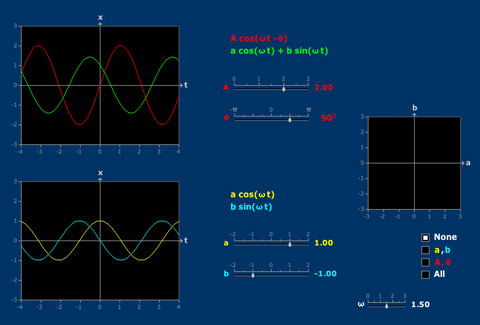# Trigonometric Identity

Any linear combination of cosine and sine (with the same frequency) is again sinusoidal. The amplitude and phase lag of the sum are related to the coefficients of cosine and sine by means of polar coordinates.Any linear combination of cosine and sine (with the same frequency) is again sinusoidal. The amplitude and phase lag of the sum are related to the coefficients of cosine and sine by means of polar coordinates.